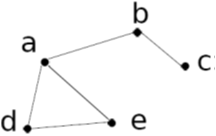# Current Slide

Small screen detected. You are viewing the mobile version of SlideWiki. If you wish to edit slides you will need to use a larger device.

### Similarity Measure (I): Geodesic Distance

• Geodesic distance (A, B): length (i.e., # of edges) of the shortest path between A and B (if not connected, defined as infinite)
• Eccentricity of v, eccen(v): The largest geodesic distance between v and any other vertex u ∈ V − {v}.
• E.g., eccen(a) = eccen(b) = 2; eccen(c) = eccen(d) = eccen(e) = 3
• Radius of graph G: The minimum eccentricity of all vertices, i.e., the distance between the “most central point” and the “farthest border”
• r = min v∈V eccen(v)
• E.g., radius (g) = 2
• Diameter of graph G: The maximum eccentricity of all vertices, i.e., the largest distance between any pair of vertices in G
• d = max v∈V eccen(v)
• E.g., diameter (g) = 3
• A peripheral vertex is a vertex that achieves the diameter.
• E.g., Vertices c, d, and e are peripheral verticesSpeaker notes:

## Content Tools

### Sources

There are currently no sources for this slide.